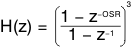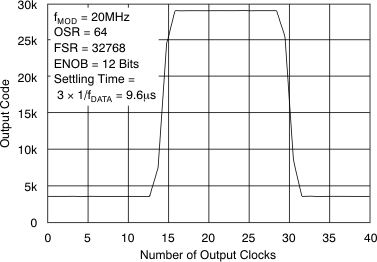SBAS512F April   2011  – February 2020

PRODUCTION DATA.

1. Features
2. Applications
3. Description
1.     Device Images
4. Revision History
5. Pin Configuration and Functions
6. Specifications
7. Detailed Description
1. 7.1 Overview
2. 7.2 Functional Block Diagram
3. 7.3 Feature Description
4. 7.4 Device Functional Modes
8. Application and Implementation
1. 8.1 Application Information
2. 8.2 Typical Application
1. 8.2.1 Frequency Inverter Application
2. 8.2.2 Example of a Resolver-Based Motor Control Analog Front End
3. 8.2.3 Isolated Voltage Sensing
9. Power Supply Recommendations
10. 10Layout
11. 11Device and Documentation Support
12. 12Mechanical, Packaging, and Orderable Information

• DWV|8
• DW|16
• DW|16

#### 8.1.1 Digital Filter Usage

The modulator generates a bit stream that is processed by a digital filter to obtain a digital word similar to a conversion result of a conventional analog-to-digital converter (ADC). A very simple filter, built with minimal effort and hardware, is a sinc3-type filter, as shown in Equation 1:

Equation 1.This filter provides the best output performance at the lowest hardware size (count of digital gates). For an oversampling rate (OSR) in the range of 16 to 256, this filter is a good choice. All the characterization in this document is also done with a sinc3 filter with OSR = 256 and an output word width of 16 bits.

In a sinc3 filter response (shown in Figure 48 and Figure 49), the location of the first notch occurs at the frequency of output data rate fDATA = fCLK/OSR. The –3-dB point is located at half the Nyquist frequency or fDATA/4. For some applications, it may be necessary to use another filter type with different frequency response. Performance can be improved, for example, by using a cascaded filter structure. The first decimation stage could be built of a sinc3 filter with a low OSR and the second stage using a high-order filter.Figure 48. Frequency Response Of The Sinc3 FilterFigure 49. Pole Response Of The Sinc3 Filter

The effective number of bits (ENOB) is often used to compare the performance of ADCs and ΔΣ modulators. Figure 51 illustrates the ENOB of the AMC1204 and AMC1204B with different oversampling ratios. In this data sheet, this number is calculated from SNR using Equation 2:

Equation 2.An example code for an implementation of a sinc3 filter in an FPGA follows. For more information, see the Combining ADS1202 with FPGA Digital Filter for Current Measurement in Motor Control Applications application note, available for download at www.ti.com.

library IEEE; use IEEE.std_logic_1164.all; use IEEE.std_logic_unsigned.all; entity FLT is port(RESN, MOUT, MCLK, CNR : in std_logic; CN5 : out std_logic_vector(23 downto 0)); end FLT; architecture RTL of FLT is signal DN0, DN1, DN3, DN5 : std_logic_vector(23 downto 0); signal CN1, CN2, CN3, CN4 : std_logic_vector(23 downto 0); signal DELTA1 : std_logic_vector(23 downto 0); begin process(MCLK, RESn) begin if RESn = '0' then DELTA1 <= (others => '0'); elsif MCLK'event and MCLK = '1' then if MOUT = '1' then DELTA1 <= DELTA1 + 1; end if; end if; end process; process(RESN, MCLK) begin if RESN = '0' then CN1 <= (others => '0'); CN2 <= (others => '0'); elsif MCLK'event and MCLK = '1' then CN1 <= CN1 + DELTA1; CN2 <= CN2 + CN1; end if; end process; process(RESN, CNR) begin if RESN = '0' then DN0 <= (others => '0'); DN1 <= (others => '0'); DN3 <= (others => '0'); DN5 <= (others => '0'); elsif CNR'event and CNR = '1' then DN0 <= CN2; DN1 <= DN0; DN3 <= CN3; DN5 <= CN4; end if; end process; CN3 <= DN0 - DN1; CN4 <= CN3 - DN3; CN5 <= CN4 - DN5; end RTL;